# November 16, 2017

## Impulse Noise Filtering - Slew Limit Filter

### Math, Programming, Impulse Noise Filtering

In the past few articles in this series I have demonstrated using a median filter to mitigate impulse noise. The problem with a median filter is that even using a small window size and taking advantage of the speed of a mostly sorted list entering an insertion sort, the filter is still significantly slower than a windowed average. There is another option. It does not filter as nicely as a median filter but does help to dampen impulse noise.

The slew rate is a measure of how quickly voltage changes over time. A windowed average acts as limiter on slew rate but usually not enough to attenuate impulse noise. However one can directly limit the slew rate of a signal. The equation is quite simple: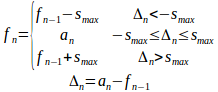For each sample of the input (an) the filter output (fn) has its change (Δn) limited to the last to some maximum (smax). If the magnitude of change is less than the maximum, the filter output is the same as the input. If the magnitude exceeds the change the filtered output is modified by the maximum change but no further.

Following the examples of previous article on this subject, here is an example of the filter output: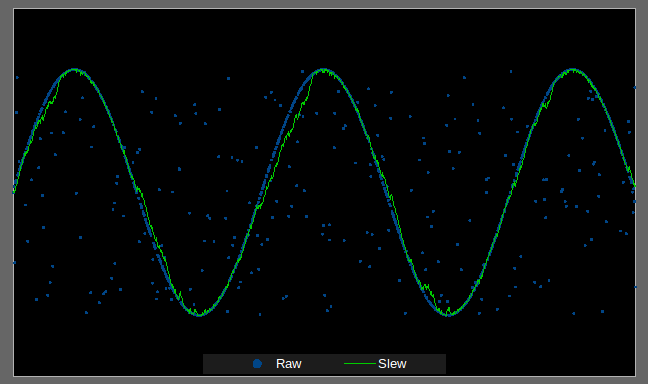There is some distortion on the signal caused by the impulse noise but the filtered output is fairly effective at eliminating the large spikes. When used in combination with a first-order low-pass filter the input signal is fairly clear.

The reason this kind of filter is attractive is because of how simple it is to implement.

//-----------------------------------------------------------------------------
// Uses:
//   In-place filter of input buffer.
// Input:
//   data - Data to filtered..
//   dataSize - Number of data points.
//   limit - Maximum change allowed.
// Output:
//   Filtered output is returned in 'data'.
// Author:
//   Andrew Que <https://www.DrQue.net/>
//-----------------------------------------------------------------------------
static inline void slewLimitFilter
(
int * data,
size_t dataSize,
int limit
)
{
for ( size_t index = 1; index < dataSize; index += 1 )
{
int delta = data[ index ] - data[ index - 1 ];
if ( delta > limit )
data[ index ] = data[ index - 1 ] + limit;
else
if ( delta < -limit )
data[ index ] = data[ index - 1 ] - limit;
}
}

Just as with any filter, artifacts are introduced by the slew limit filter.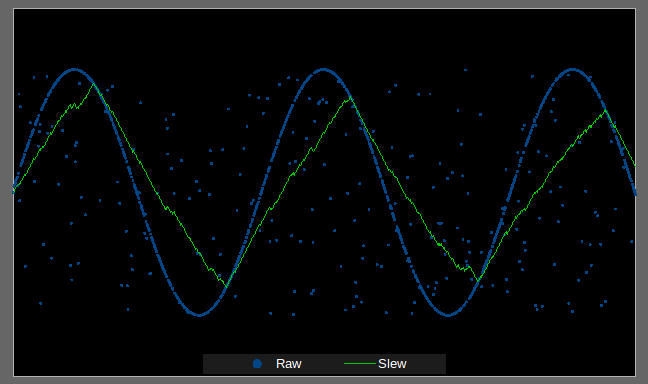This sine wave has become a triangle wave because the slew limit is too low. This shape is the result of the filter attenuating the actual data signal. This is an other demonstration.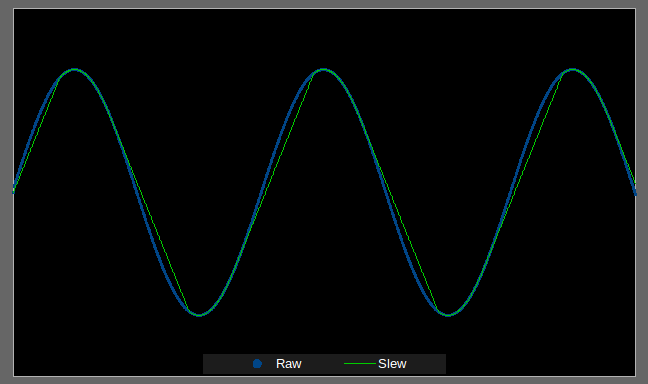Here is a clean signal, but because the slew limit is too low the filter cannot keep up with the rate of change of the true signal.

The other problem with the slew limit filter is that it is not very strong.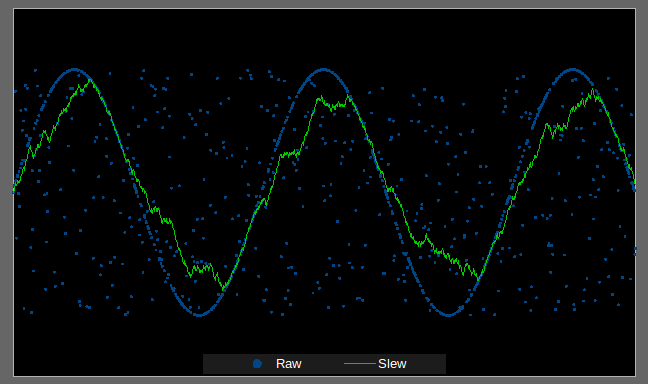Here the impulse noise has been doubled and the filter has produced a very messy result. So the filter is clearly not a replacement for a median filter. If you can afford the processing power, use the median filter. If you cannot, the slew limit filter may be a possibility. It depends on what the impulse noise looks like, and how much signal distortion can be tolerated.

# October 11, 2017

## Examining Old Algorithms

### Math

Back in the late 1990s I was working on a game inspired by the old BBS game Trade Wars 2002. I was looking to have a more advanced value system for traded goods. The other day I was interested in the algorithm I had come up with because I knew it was rather strange. So I decided to find the code and graph the value function I had created. Here is a graph of the function I created.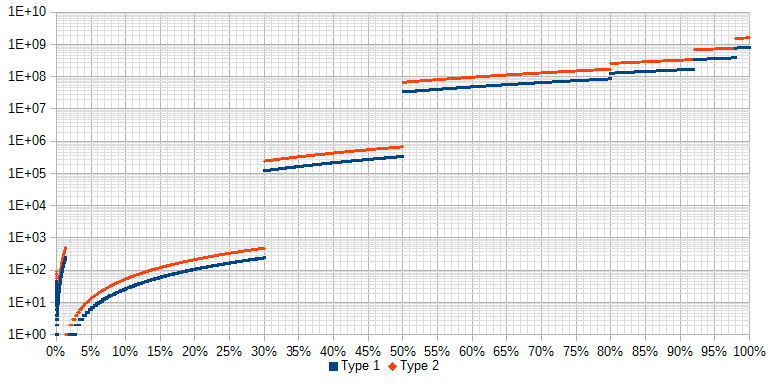The purpose of the algorithm was to produce a monetary value based on several attributes that summed to an overall quality rank. Think of this like a gemstone where 100% would be completely flawless and 0% complete flawed. One of the attribute flags could change the value by a scale factor.

When I wrote this algorithm I didn’t know much about the curving numbers. I setup a kind of liner piece-wise function. Six of the steps are fairly obvious: 30, 50, 80, 92 and 98. Probably less intentional were the last two steps. The first happens at 19/32768, and the second 448/32768. My goal was likely to make the slope toward zero more gradual near the end, but since I didn’t understand graphing a function the actual results were not at all what was expected.

The graph is shown on a logarithmic scale so the nuances like the strange end are clearly visible. All the values are actually linear and the curves of (like the one from 2% to 30%) is just a result of the logarithmic scale.

If I were to write this algorithm today I probably would use a second degree polynomial or an exponential function. Something like: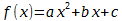or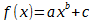By varying the coefficients a, b, and c I would have a continuous function that curved. The exponential function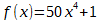will produce the following graph which is fairly close to what the game would have needed.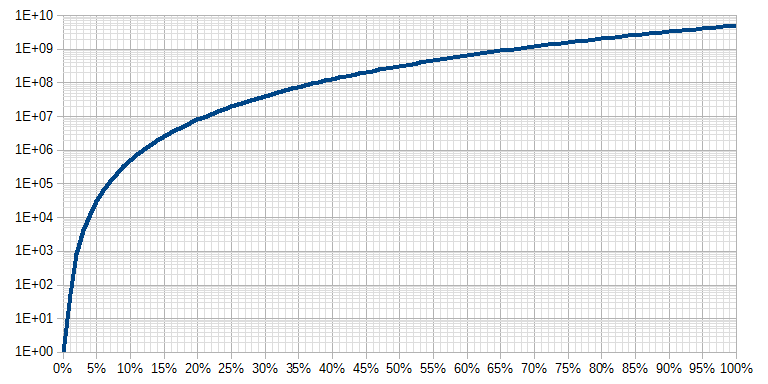Like most of my programming projects the game never amounted to much more than a concept. I spent more time fighting with the 640 KiB barrier at the time than doing much development work. It is interesting to look at how I approached math problems when I didn’t know any formal math outside of arithmetic.

# January 12, 2017

## Orthogonal Function with Dot Product Test

### Math

Playing some more with orthogonal functions to try and get a better handle of why a Fourier transform works. I decided I would try some simple functions I can work with easily to test orthogonality. The definition for as orthogonal function is one such that: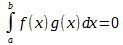So I decided to use two very simple functions over the interval b = -a.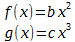Orthogonal test: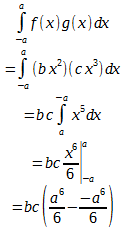Remember that ab even power b is the same for a and -a. Thus: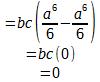So my two functions are orthogonal. Now for something crazy.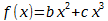I want to extract the coefficient for b. So I can to a projection for x2.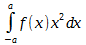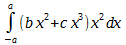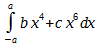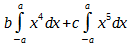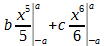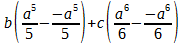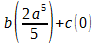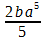Notice how c does not show up in this result.

The same for x3.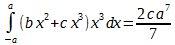Here, b does not show up in the result.

I verified this using a discrete check in a speadsheet. While I expected it would work, watching it actually do so was interesting.

# January 05, 2017

## FFT for LibreOffice Calc

### Math, Programming

A few days ago I posted a ooBasic script that can be used in LibreOffice to do a Discrete Fourier Transform (DFT) on a set of cells. While it works, it is slow. This is because the a raw implementation of a discrete Fourier transform runs at a complexity of O( n2 ). That is, the number of operations required to compute is the square of the number of data points. Since this grows exponentially, slightly larger data sets take significantly longer to compute.

Naturally, the solution is to switch to a Fast Fourier Transform (FFT). These can generally reduce the complexity down to O( n log2 n ). I knew this when implementing a DFT, but implementing an FFT is a bit more complected. ooBasic kinda...well, pretty much sucks as a programing language. So to do an FFT implementation I wanted an algorithm fairly straight forward to translate. I surveyed several libraries but ultimately decided on Free small FFT. It included a fairly clean radix-2 Cooley–Tukey FFT algorithm but also had Bluestein's algorithm for data not in powers-of-two. The complexity looks to be something around: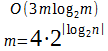I am judging this based on the fact it does three radix-2 transforms on a four times the rounded up power-of-two.

Why does anyone need an FFT in a spreadsheet? I’m not the only person who has searched for such a function, but the primary reason I want one is to do a spectrum plot of a sampled signal. It doesn’t happen often that I need one, but when I want it, I want it in the spreadsheet and would rather not have to go to an external tool.

Today I finished making a simple project page to host the script. Installing it in LibreOffice isn’t easy, but the steps outline in the manual section seem to work. They don’t make it easy, but should anyone else ever want LibreOffice Calc to do an FFT, this should work.